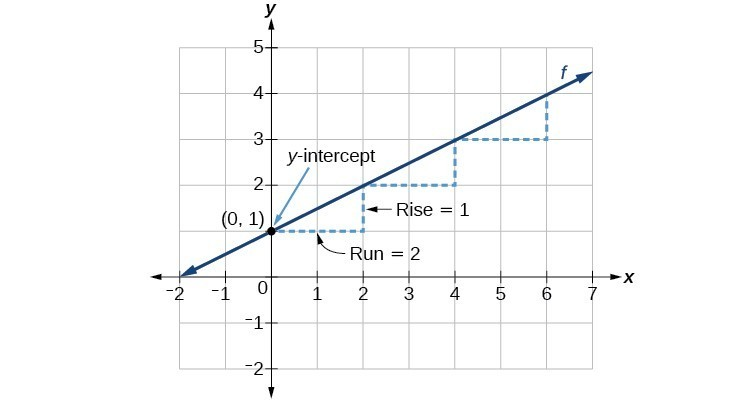## Graph Linear Functions Using Slope and y-Intercept

### Learning Outcome

• Graph a linear function using the slope and y-intercept

Another way to graph a linear function is by using its slope and y-intercept.

Let us consider the following function.

$f\left(x\right)=\dfrac{1}{2}x+1$

The function is in slope-intercept form, so the slope is $\dfrac{1}{2}$. Because the slope is positive, we know the graph will slant upward from left to right. The y-intercept is the point on the graph when $x=0$. The graph crosses the y-axis at $(0, 1)$. Now we know the slope and the y-intercept. We can begin graphing by plotting the point $(0, 1)$. We know that the slope is rise over run, $m=\dfrac{\text{rise}}{\text{run}}$. From our example, we have $m=\dfrac{1}{2}$, which means that the rise is $1$ and the run is $2$. So starting from our y-intercept $(0, 1)$, we can rise $1$ and then run $2$, or run $2$ and then rise $1$. We repeat until we have a few points and then we draw a line through the points as shown in the graph below.### A General Note: Graphical Interpretation of a Linear Function

In the equation $f\left(x\right)=mx+b$

• b is the y-intercept of the graph and indicates the point (0, b) at which the graph crosses the y-axis.
• m is the slope of the line and indicates the vertical displacement (rise) and horizontal displacement (run) between each successive pair of points. Recall the formula for the slope:

$m=\dfrac{\text{change in output (rise)}}{\text{change in input (run)}}=\dfrac{\Delta y}{\Delta x}=\dfrac{{y}_{2}-{y}_{1}}{{x}_{2}-{x}_{1}}$

All linear functions cross the y-axis and therefore have y-intercepts. (Note: A vertical line parallel to the y-axis does not have a y-intercept, but it is not a function.)

### How To: Given the equation for a linear function, graph the function using the y-intercept and slope

1. Evaluate the function at an input value of zero to find the y-intercept.
2. Identify the slope.
3. Plot the point represented by the y-intercept.
4. Use $\dfrac{\text{rise}}{\text{run}}$ to determine at least two more points on the line.
5. Sketch the line that passes through the points.

### Example

Graph $f\left(x\right)=-\dfrac{2}{3}x+5$ using the y-intercept and slope.

### Try It

In the following video we show another example of how to graph a linear function given the y-intercepts and the slope.

In the last example, we will show how to graph another linear function using the slope and y-intercept.

### Example

Graph $f\left(x\right)=-\dfrac{3}{4}x+6$ using the slope and y-intercept.